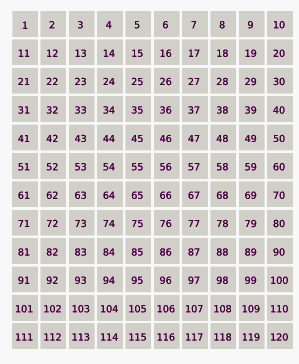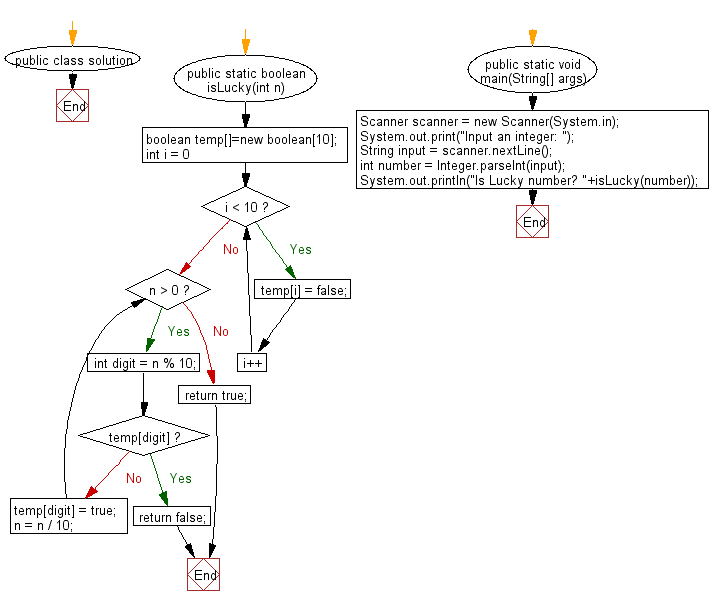﻿ Java exercises: Check whether a number is a Lucky Number or not - w3resource# Java Exercises: Check whether a number is a Lucky Number or not.

## Java Numbers: Exercise-29 with Solution

Write a Program in Java to check whether a number is a Lucky Number or not.

Lucky numbers are defined via a sieve as follows.
Begin with a list of integers starting with 1 :
1, 2, 3, 4, 5, 6, 7, 8, 9, 10, 11, 12, 13, 14, 15, 16, 17, 18, 19, 20, 21, 22, 23, 24, 25, . . . .
Now eliminate every second number :
1, 3, 5, 7, 9, 11, 13, 15, 17, 19, 21, 23, 25, ...
The second remaining number is 3, so remove every 3rd number:
1, 3, 7, 9, 13, 15, 19, 21, 25, ...
The next remaining number is 7, so remove every 7th number:
1, 3, 7, 9, 13, 15, 21, 25, ...
Next, remove every 9th number and so on.
Finally, the resulting sequence is the lucky numbers.

Following animation demonstrating the lucky number sieve. The numbers in red are lucky numbers.Animation Credits: Celtic Minstrel

Sample Solution:

Java Code:

``````import java.util.*;
public class solution {
public static boolean isLucky(int n) {
//Create an array of size 10 to initialize all elements as
//false to check if a digit is already seen or not.
boolean temp[]=new boolean;
for (int i = 0; i < 10; i++)
temp[i] = false;
while (n > 0)
{
// Find the last digit
int digit = n % 10;
// Return false if digit is already seen,
if (temp[digit])
return false;
temp[digit] = true;
n = n / 10;
}
return true;
}

public static void main(String[] args) {
Scanner scanner = new Scanner(System.in);
System.out.print("Input an integer: ");
String input = scanner.nextLine();
int number = Integer.parseInt(input);
System.out.println("Is Lucky number? "+isLucky(number));
}
}
```
```

Sample Output:

```Input an integer:  25
Is Lucky number? true
```

Flowchart:Java Code Editor:

What is the difficulty level of this exercise?

﻿

## Java: Tips of the Day

Array vs ArrayLists:

The main difference between these two is that an Array is of fixed size so once you have created an Array you cannot change it but the ArrayList is not of fixed size. You can create instances of ArrayLists without specifying its size. So if you create such instances of an ArrayList without specifying its size Java will create an instance of an ArrayList of default size.

Once an ArrayList is full it re-sizes itself. In fact, an ArrayList is internally supported by an array. So when an ArrayList is resized it will slow down its performance a bit as the contents of the old Array must be copied to a new Array.

At the same time, it's compulsory to specify the size of an Array directly or indirectly while creating it. And also Arrays can store both primitives and objects while ArrayLists only can store objects.

Ref: https://bit.ly/3o8L2KH